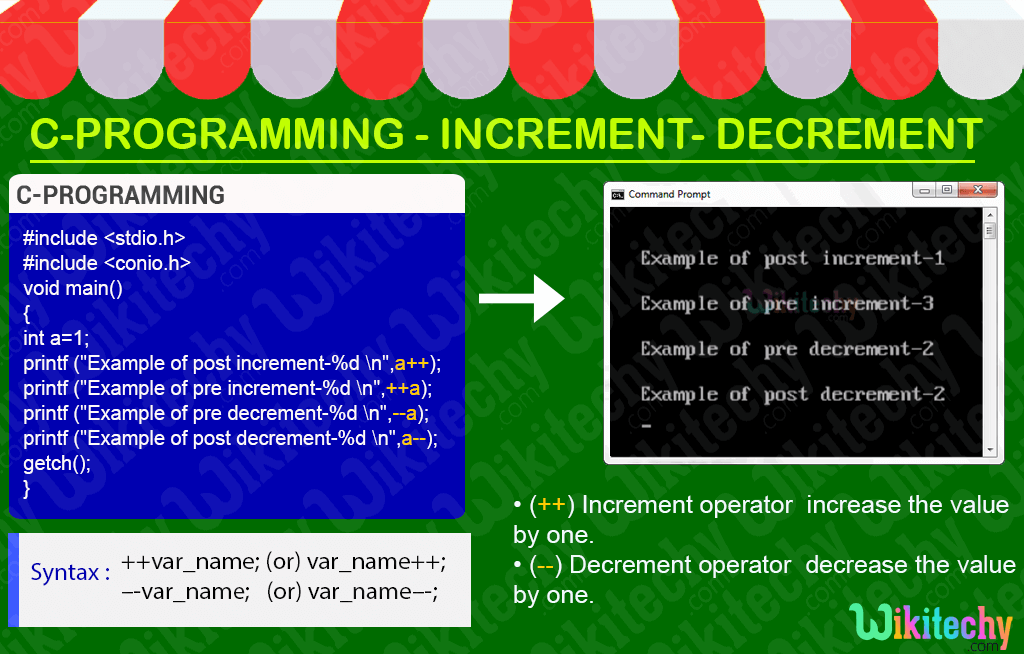# Operator in C

## C - Operator - Definition and Usage

• In C-Programming the symbols are used for logical and mathematical operations called as operators in C.
• Types of operators in C-programming :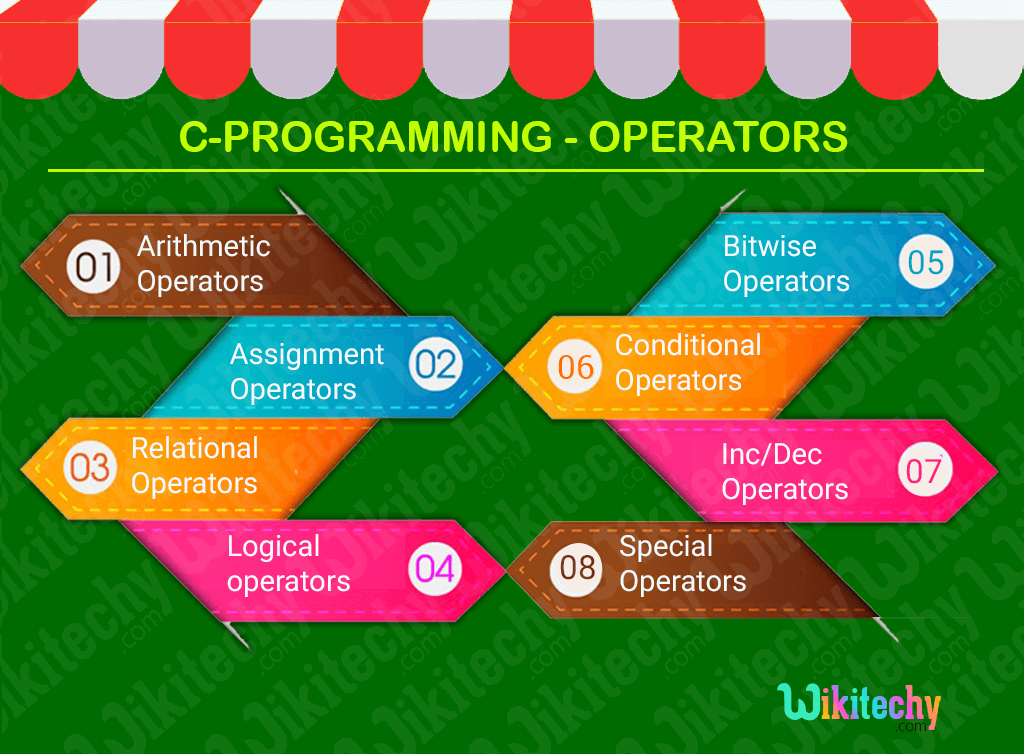## C Arithmetic operators - Definition and Usage

• In C-Programming the Arithmetic operators is used to perform mathematical calculation such as
• Subtraction(-),
• Multiplication(*),
• Division(/) and
• Modulus(%).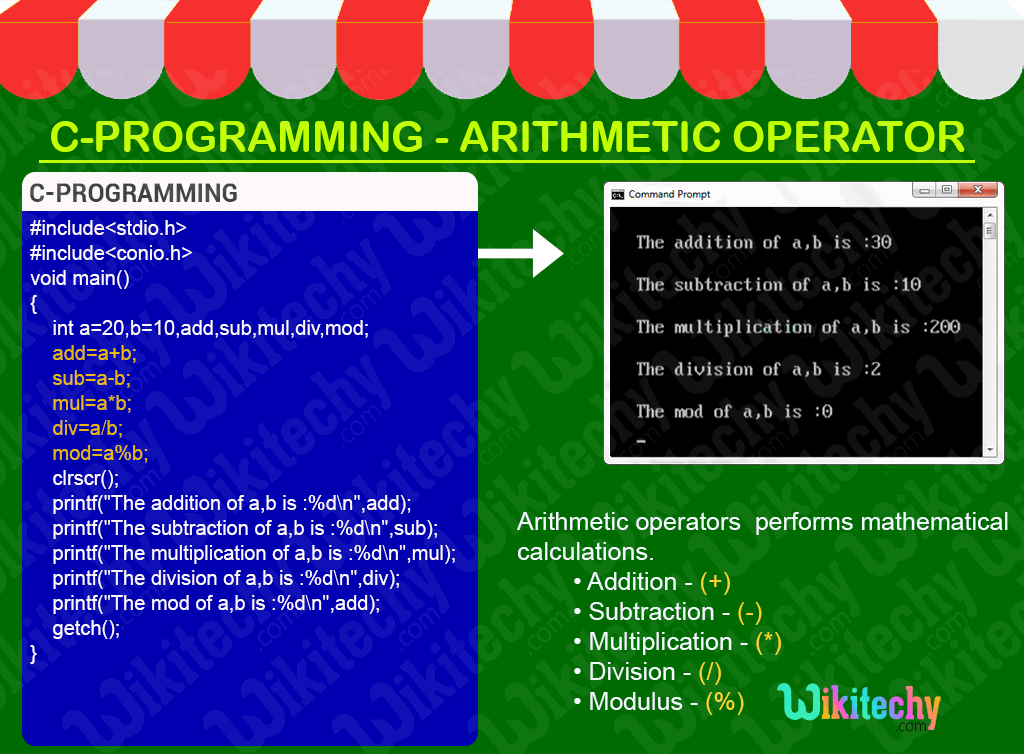## C Assignment operator - Definition and Usage

• In C-program the assignment operator is used for assigning a value to a variable.
• The most common assignment operator is = (equals to).
• The assignment operators tend to be classified into two categories such as,
• Simple assignment operator.
• Compound assignment operator.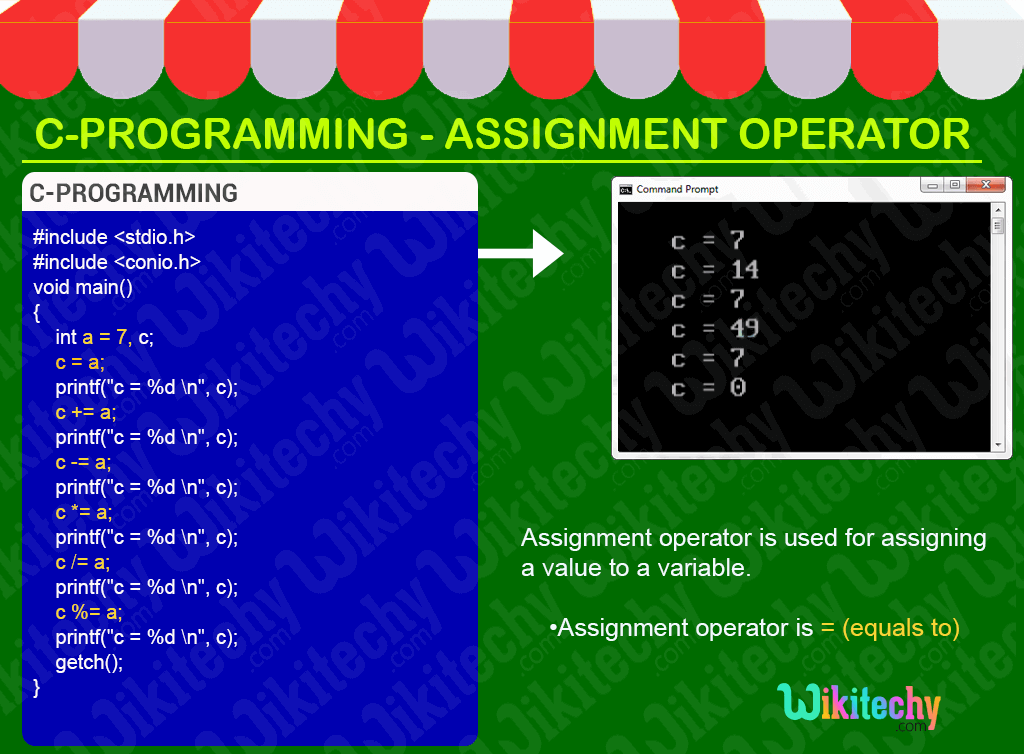## C Logical operator- Definition and Usage

• In C-Programming the logical operators are used for defining the logical operation.
• Generally, there are three logical operators in c language.
• logical AND (&&)
• logical OR (||)
• logical NOT (!)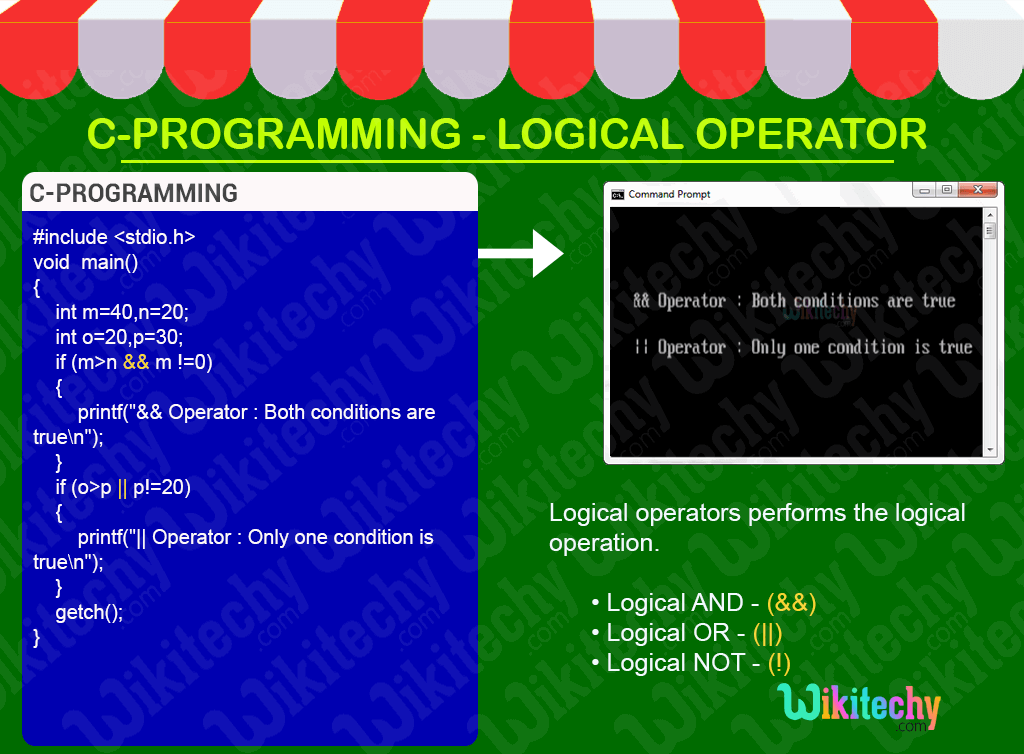## C Bitwise Operator - Definition and Usage

• In C-programming the bitwise operators are used to perform bit operations.
• That is the decimal values are converted into binary values which are the sequence of bits and bit wise operators work on these bits.
• Bit wise operators in C programming are
• & (bitwise AND),
• | (bitwise OR),
• ~ (bitwise NOT),
• ^ (bitwise XOR),
• << (bitwise left shift) and
• >> (bitwise right shift).## C Conditional Operator - Definition and Usage

• In C-programming the conditional operator return one value if condition is true and returns another value if condition is false.
• Conditional operators is also called as ternary operator.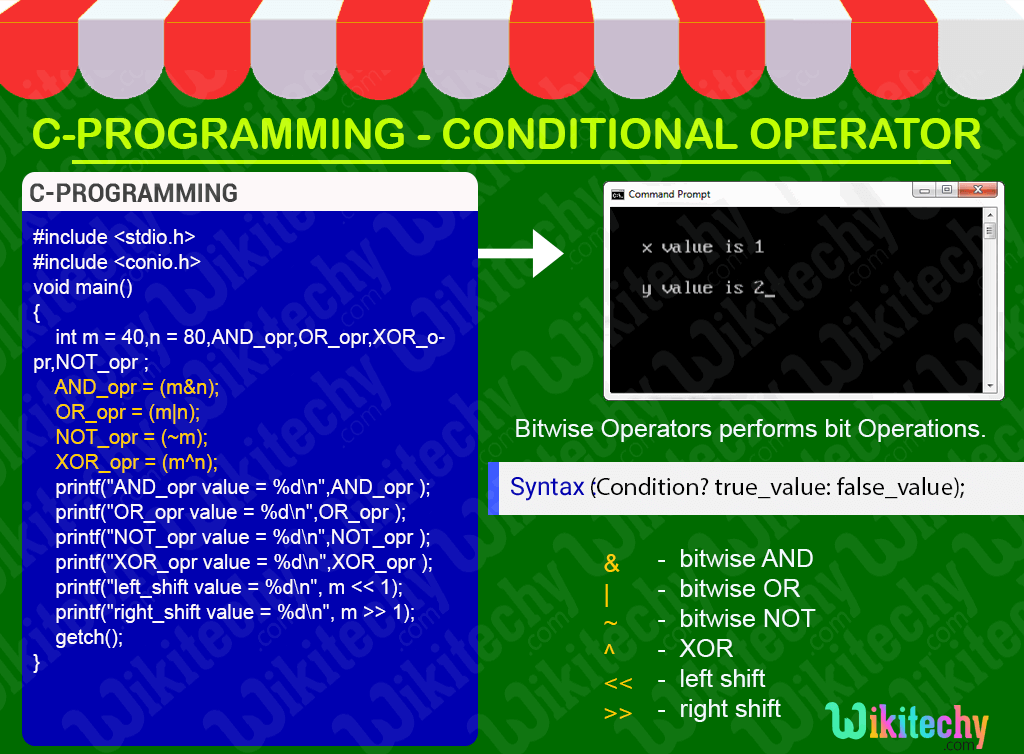## C Special Operator - Definition and Usage

• In C-programming the special operators are mostly used for memory related functions.
• In C-language some special operators are listed below as follows:
• & Operator is used to get the address of the variable;
• * Operator is used as pointer of the variable.
• Sizeof() Operator is used to define the size of the variable## C Increment/Decrement Operator - Definition and Usage

• In C- Programming Increment operator (++) is used to increase the value of the variable by one .
• In C- Programming decrement operator (--) is used to decrease the value of the variable by one .